# The Universe of Discourse

Thu, 15 Dec 2016

The Parshvanatha temple in Madhya Pradesh, India was built around 1,050 years ago. Carved at its entrance is this magic square: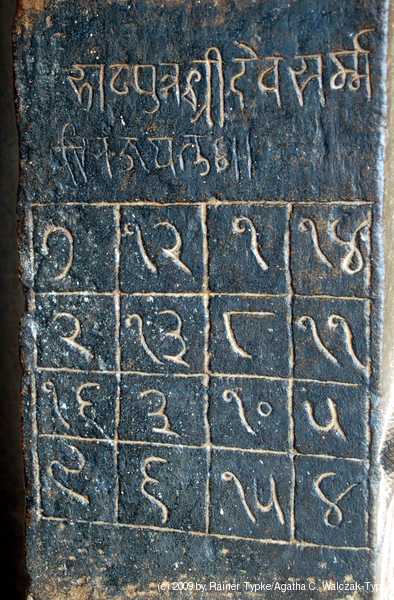The digit signs have changed in the past thousand years, but it's a quick and fun puzzle to figure out what they mean using only the information that this is, in fact, a magic square.

A solution follows. No peeking until you've tried it yourself!

There are 9 one-digit entries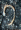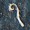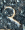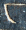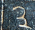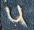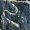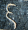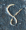and 7 two-digit entries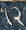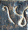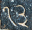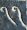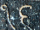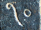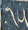so we can guess that the entries are the numbers 1 through 16, as is usual, and the magic sum is 34. Theappears in the same position in all the two-digit numbers, so it's the digit 1. The other digit of the numeralis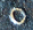, and this must be zero. If it were otherwise, it would appear on its own, as does for example thefromor thefrom.

It is tempting to imagine thatis 4. But we can see it's not so. Adding up the rightmost column, we get+++=+ 11 ++=
(10 +) + 11 ++= 34,

so thatmust be an odd number. We know it isn't 1 (becauseis 1), and it can't be 7 or 9 becauseappears in the bottom row and there is no 17 or 19. Somust be 3 or 5.

Now ifwere 3, thenwould be 13, and the third column would be+++=
1 ++ 10 + 13 = 34,

and thenwould be 10, which is too big. Somust be 5, and this means thatis 4 andis 8. (appears only a as a single-digit numeral, which is consistent with it being 8.)

The top row has+++=++ 1 + 14 =+ (10 +) + 1 + 14 = 34

so that+= 9.only appears as a single digit and we already used 8 somust be 7 or 9. But 9 is too big, so it must be 7, and thenis 2.is the only remaining unknown single-digit numeral, and we already know 7 and 8, sois 9. The leftmost column tells us thatis 16, and the last two entries,andare easily discovered to be 13 and 3. The decoded square is:7 12 1 14 2 13 8 11 16 3 10 5 9 6 15 4

I like that people look at the right-hand column and immediately see 18 + 11 + 4 + 8 but it's actually 14 + 11 + 5 + 4.

This is an extra-special magic square: not only do the ten rows, columns, and diagonals all add up to 34, so do all the four-cell subsquares, so do any four squares arranged symmetrically about the center, and so do all the broken diagonals that you get by wrapping around at the edges.

[ Addendum: It has come to my attention that the digit symbols in the magic square are not too different from the current forms of the digit symbols in the Gujarati script. ]

[ Addendum 20161217: The temple is not very close to Gujarat or to the area in which Gujarati is common, so I guess that the digit symbols in Indian languages have evolved in the past thousand years, with the Gujarati versions remaining closest to the ancient forms, or else perhaps Gujarati was spoken more widely a thousand years ago. I would be interested to hear about this from someone who knows. ]

[ Addendum 20170130: Shreevatsa R. has contributed a detailed discussion of the history of the digit symbols. ]